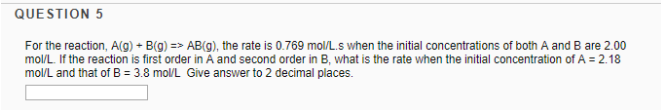# Problem: For the reaction, A(g) + B(g) → AB(g), the rate is 0.769 mol/Ls when the initial concentrations of both A and B are 2.00 mol/L. If the reaction is first order in A and second order in B, what is the rate when the initial concentration of A = 2.18 mol/L and that of B = 3.8 mol/L Give answer to 2 decimal places.

###### FREE Expert Solution
92% (183 ratings)###### Problem Details

For the reaction, A(g) + B(g) → AB(g), the rate is 0.769 mol/Ls when the initial concentrations of both A and B are 2.00 mol/L. If the reaction is first order in A and second order in B, what is the rate when the initial concentration of A = 2.18 mol/L and that of B = 3.8 mol/L Give answer to 2 decimal places.